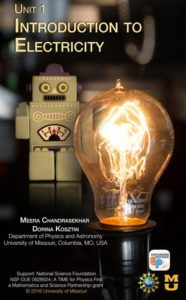### Unit 1 Introduction to Electricity        View Unit 1This is an introductory unit to electricity. Students begin the unit by experiencing static electricity and then creating simple circuits. Utilizing buzzers, motors, bulbs, switches, and multimeters, students devise a model of current and measure voltage. By the end of the unit, students will be able to answer the following questions:

• What happens when objects get charged?
• What does it take to light a bulb?
• What materials conduct electric current?
• Is there a direction in which electric current flows?
• How does a switch in a circuit work?
• How do LEDs and photoresistors work?
• What does it take to make a battery?

Buy materials for teaching Unit 1 hands-on labs.

Suggested timeline for teaching Unit 1

Big Ideas
1. A completed path must be provided for electric current to flow.
2. For charges to move a voltage must be provided.

Learning Goals and Objectives
By the end of this unit, the students should be able to:

1. Investigate working electrical circuits. (DOK3) a) Describe types of electrical charges and their interactions. b) Describe how electrical devices work. c) Identify the contact points for batteries, bulbs, and switches. d) Explain how batteries, bulbs, and switches work. e) Design and conduct an investigation to distinguish between conductors and insulators. f) Distinguish between open and closed circuits. g) Provide evidence that current flows in a specific direction. h) Use a multimeter to measure current, voltage and resistance.
2. Describe an electrical circuit, using multiple representations (i.e., pictorial diagrams, verbal descriptions, schematics, and mathematical models, etc.). (DOK3) a) Create a verbal, schematic, or pictorial description of a physical electrical circuit. b) Construct an electrical circuit from the verbal, schematic, or pictorial representation of a circuit.
3. Design and conduct an experiment to determine how different electrodes and electrolytes affect the voltage produced by a wet-cell battery. (DOK4) a) Define voltage and measure a battery’s voltage. b) Identify the essential components of a wet-cell battery. c) Construct batteries using different combinations of electrodes and electrolytes. d) Measure the voltage of the batteries constructed.
4. Design and conduct experiments to study parameters that affect electrical circuits. (DOK4) a) Make observations. b) Operationally define the variables. c) Generate a hypothesis.